# Basic Arithmetic : Mixed Numbers

## Example Questions

### Example Question #1 : Mixed Numbers

What is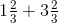in fraction form?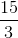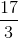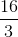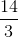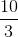Explanation:

To rewrite the mixed fractions as improper fractions we multiply the whole number by the denominator and add the numerator to get our new numerator. Our denominator stays the same. With this in mind we get the following: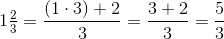and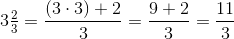.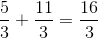.

### Example Question #1 : Manipulation Of Fractions

Convert this mixed number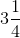to an improper fraction.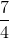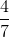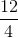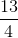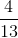Explanation:

The correct answer is.

The mixed number can be broken down as follows: 3 is the whole number, 1 is the numerator, and 4 is the denominator.

In order to convert the mixed number to an improper fraction, you must first multiply the denominator with the whole number and then add the numerator to it.

This answer is the numerator of the improper fraction: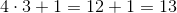.

The denominator of the fraction remains the same and the final answer is.

### Example Question #2 : Manipulation Of Fractions

What is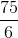equivalent to?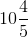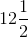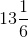Explanation:

This question is asking you to change an improper fraction into a mixed number. To do that we will need to divide 75 by 6.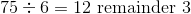Now, we place the remainder as the numerator of a fraction with a denominator that is 6 because we just divided by six.

So then, 12 remainder 3 becomes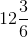.

Simplify this mixed fraction.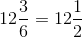### Example Question #1 : Manipulation Of Fractions

Find the sum.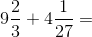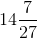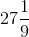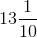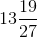Explanation:

To add mixed numbers, you need to first change both of them into improper fractions.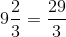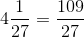Then, add the two improper fractions like you would any other fraction.

We need both fractions to have the same denominator before we can add them. Since 27 is a multiple of 3, we only need to change 1 fraction.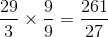Now, we can add these fractions.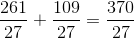Finally, change the improper fraction back into a mixed number.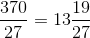### All Basic Arithmetic Resources